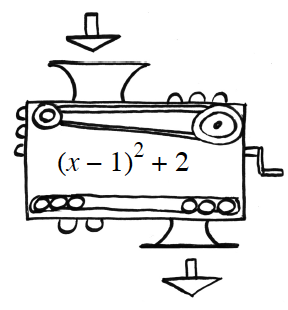### Home > INT2 > Chapter 9 > Lesson 9.1.2 > Problem9-17

9-17.Carmen made a function machine. The inner workings of the machine are visible in the diagram at right. What will the output be in each of the following cases?

1. If $2$ is dropped in?

Substitute $2$ for every $x$ (into ($x - 1$)$^2+ 2$).
($2 - 1$)$^2+ 2$

$3$

2. If $- 4$ is dropped in?

Substitute ($-4$) for every $x$ in the expression.

3. If $-11.3$ is dropped in?

$153.29$